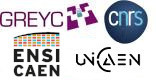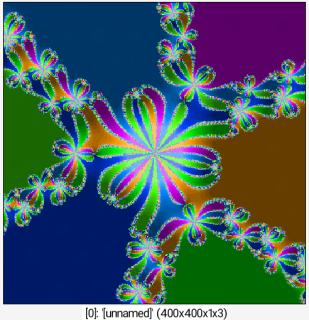G'MIC - GREYC's Magic for Image Computing: A Full-Featured Open-Source Framework for Image ProcessingReference

# newton_fractal

## Arguments:

• z0r,z0i,z1r,z1i,_angle,0<=_descent_method<=2,_iteration_max>=0,_convergence_precision>0,_expr_p(z),_expr_dp(z),_expr_d2p(z)

## Description:

Draw newton fractal on selected images, for complex numbers in range (z0r,z0i) - (z1r,z1i).

Resulting images have 3 channels whose meaning is [ last_zr, last_zi, nb_iter_used_for_convergence ].
descent_method can be { 0=secant | 1=newton | 2=householder }.

## Default values:

angle=0, descent_method=1, iteration_max=200, convergence_precision=0.01, expr_p(z)=z^^3-1, expr_dp(z)=3*z^^2 and expr_d2z(z)=6*z.

## Example of use:

\$ gmic 400,400 newton_fractal -1.5,-1.5,1.5,1.5,0,2,200,0.01,"z^^6 + z^^3 - 1","6*z^^5 + 3*z^^2","30*z^^4 + 6*z" f "[ atan2(i1,i0)*90+20,1,cut(i2/30,0.2,0.7) ]" hsl2rgbCommand: 400,400 newton_fractal -1.5,-1.5,1.5,1.5,0,2,200,0.01,"z^^6 + z^^3 - 1","6*z^^5 + 3*z^^2","30*z^^4 + 6*z" f "[ atan2(i1,i0)*90+20,1,cut(i2/30,0.2,0.7) ]" hsl2rgb
G'MIC - GREYC's Magic for Image Computing: A Full-Featured Open-Source Framework for Image Processing